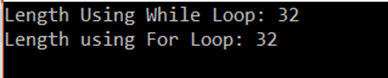AWS Global Infrastructure

### Programming & Frameworks

Topics Covered
• C Programming and Data Structures (26 Blogs)
• Comprehensive Java Course (1 Blogs)
• Java/J2EE and SOA (321 Blogs)
• Spring Framework (8 Blogs)
SEE MORE

# How To Calculate String Length In C++?

Last updated on Aug 08,2019 2.5K ViewsHow To Calculate String Length In C++?

There will be occasions when you will required to work strings of different length while you program. This article will tell you how calculate string length in C++.

A String is a character array. It is a sequential collection of characters or an array of characters. Length of a string is essential while coding with strings There are many methods to find the length of the string. We would be discussing three out of those

## Using a Loop:

A loop is used with a count variable to find the length of the string.

## Let’s look at the code:

```#include<iostream>
using namespace std;
int main()
{
string str = "Finding The Length Of The String";
int i = 0,count=0;
while (str[i] != '')
{
++i;
}
cout <<"Length Using While Loop: "<< i << endl;
for (i=0; str[i]!=''; i++)
{
count++;
}
cout <<"Length using For Loop: "<< count << endl;
return 0;
}
```

## Output:## Explanation:

In the above program, we find the length of the string using 2 loops. A While loop and a For loop. We first have a string “Finding The Length Of The String”. Followed by i and count variable that is initialized to 0.

Every string end’s with a ‘/0′. It is called an escape sequence. It is not a special character but a literal number zero.

The while loop runs till the str[i] is not equal to the escape sequence.

```while(str[i]!='')
{
++i;
}
```

Inside the While loop, the value of i is incremented from zero, till the last character of the string is found. This gives the length of the string.

Next, we have the for loop.

```for (i=0; str[i]!=''; i++)
{
count++;
}
```

The for loop runs from i=0 till the end character is encountered. Inside, the count variable is incremented every time until we reach the end of the string. We print the value of count. We can also just print the value of i as well as it will also give the length of the string.

## Using an in-built function:

There are two in-built functions, size() and length(). Both these functions give the same result when they are used. The return length of the string in terms of bytes.

## Let’s look at the code:

```#include<iostream>
using namespace std;
int main()
{
string str = "Finding The Length Of The String";
cout <<"The Length of the String using length() function: "<< str.size() << endl;
cout<<"The Length of the String using length() function: "<< str.length() << endl;
return 0;
}
```

## Output:## Explanation:

In the above program, finding the length of the string is quite easier. It is done by simply calling the in-built function called size() and length(), both returning the same value.

## Using a C- Style function:

We can find the length of the string using a strlen function. This method is complex is not recommended to use. In this method, we use the string.h header file and use strlen function in it.

## Let’s look at the code:

```#include<iostream>
using namespace std;
int main()
{
string str = "Finding The Length Of The String";
cout<<"String Length is: "<< strlen(str.c_str()) << endl;
return 0;
}
```

## Output:## Explanation:

In the above program, finding the length of the string is a bit complex. We first need to include the string.h header file. The next step is,

`strlen(str.c_str())`

We call the strlen function and tell the compiler that str is of the old string style. Then a simple strlen function executes like how it does in c.

## These are the three ways to get the string length.

Thus we have come to an end of this article on ‘String Length in C++’. If you wish to learn more, check out the Java Training by Edureka, a trusted online learning company. Edureka’s Java J2EE and SOA training and certification course is designed to train you for both core and advanced Java concepts along with various Java frameworks like Hibernate & Spring.

Got a question for us? Please mention it in the comments section of this blog  and we will get back to you as soon as possibleREGISTER FOR FREE WEBINARThank you for registering Join Edureka Meetup community for 100+ Free Webinars each month